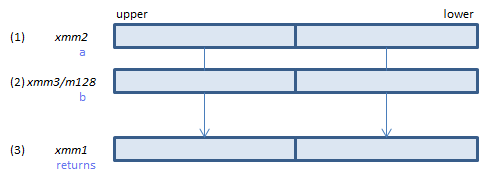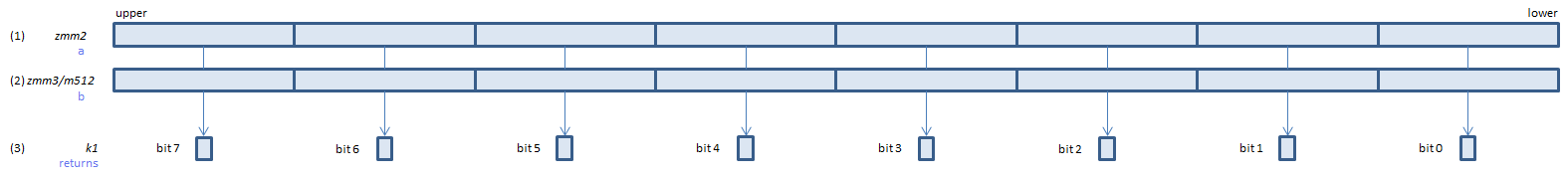﻿ vcmptrue_uspd

## VCMPTRUE_USPD - CoMPare TRUE Unordered Signal Packed Double

VCMPTRUE_USPD xmm1, xmm2, xmm3/m128    (V1
__m128d _mm_cmp_pd(__m128d a, __m128d b, _CMP_TRUE_US)For each double, always set 1 to all bits of the corresponding double of (3).
VCMPTRUE_USPD ymm1, ymm2, ymm3/m256    (V1
__m256d _mm256_cmp_pd(__m256d a, __m256d b, _CMP_TRUE_US)For each double, always set 1 to all bits of the corresponding double of (3).
VCMPTRUE_USPD k1{k2}, xmm2, xmm3/m128/m64bcst    (V5+VLFor each double, always set 1 to the corresponding bit of (3).
If k2 bit is 0, the comparison is not done and the corresponding bit of (3) is set to zero. Upper bits of (3) are zero cleared.
VCMPTRUE_USPD k1{k2}, ymm2, ymm3/m256/m64bcst    (V5+VLFor each double, always set 1 to the corresponding bit of (3).
If k2 bit is 0, the comparison is not done and the corresponding bit of (3) is set to zero. Upper bits of (3) are zero cleared.
VCMPTRUE_USPD k1{k2}, zmm2, zmm3/m512/m64bcst{sae}    (V5